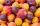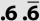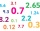# Closest natural number

Find the closest natural number to 4.456 to 44.56 and to 445.6.

Result

a =  4
b =  45
c =  446

#### Solution:Leave us a comment of example and its solution (i.e. if it is still somewhat unclear...):

Showing 0 comments:Be the first to comment!## Next similar examples:

1. Roman numerals 2-Subtract up the number written in Roman numerals. Write the results as Roman numbers.
2. Number with onesThe first digit of the number is 1, if we move this digit to the end we get a 3 times higher number, which is the number?
3. Write decimalsWrite in the decimal system the short and advanced form of these numbers: a) four thousand seventy-nine b) five hundred and one thousand six hundred and ten c) nine million twenty-six
4. HomeworkIn the crate are 18 plums, 27 apricot and 36 nuts. How many pieces of fruit left in the crate when Peter took 8 ninth: 1. nuts 2. apricots 3. fruit 4. drupe
5. RoundingDouble round number 727, first to tens, then to hundreds. (double rounding)
6. Valid numberRound the 453874528 on 2 significant numbers.
7. To improper fractionChange mixed number to improper fraction a) 1 2/15 b) -2 15/17
8. A numberA number increased by 7.9 is 8.3
9. RoundingThe following numbers round to the thousandth:
10. What is one thirdWhat is 1/3 as a decimal? Give your answer rounded to 2 decimal places.
11. Equation 11Solve equation: ?
12. FractionDetermine for what x fraction ?:
13. Round it0.728 round to units, tenths, hundredths.
14. Negative in equation2x + 3 + 7x = – 24, what is the value of x?
15. Passenger boatTwo-fifths of the passengers in the passenger boat were boys. 1/3 of them were girls and the rest were adult. If there were 60 passengers in the boat, how many more boys than adult were there?
16. NormThree workers planted 3555 seedlings of tomatoes in one dey. First worked at the standard norm, the second planted 120 seedlings more and the third 135 seedlings more than the first worker. How many seedlings were standard norm?
17. Simple equationSolve the following simple equation: 2. (4x + 3) = 2-5. (1-x)